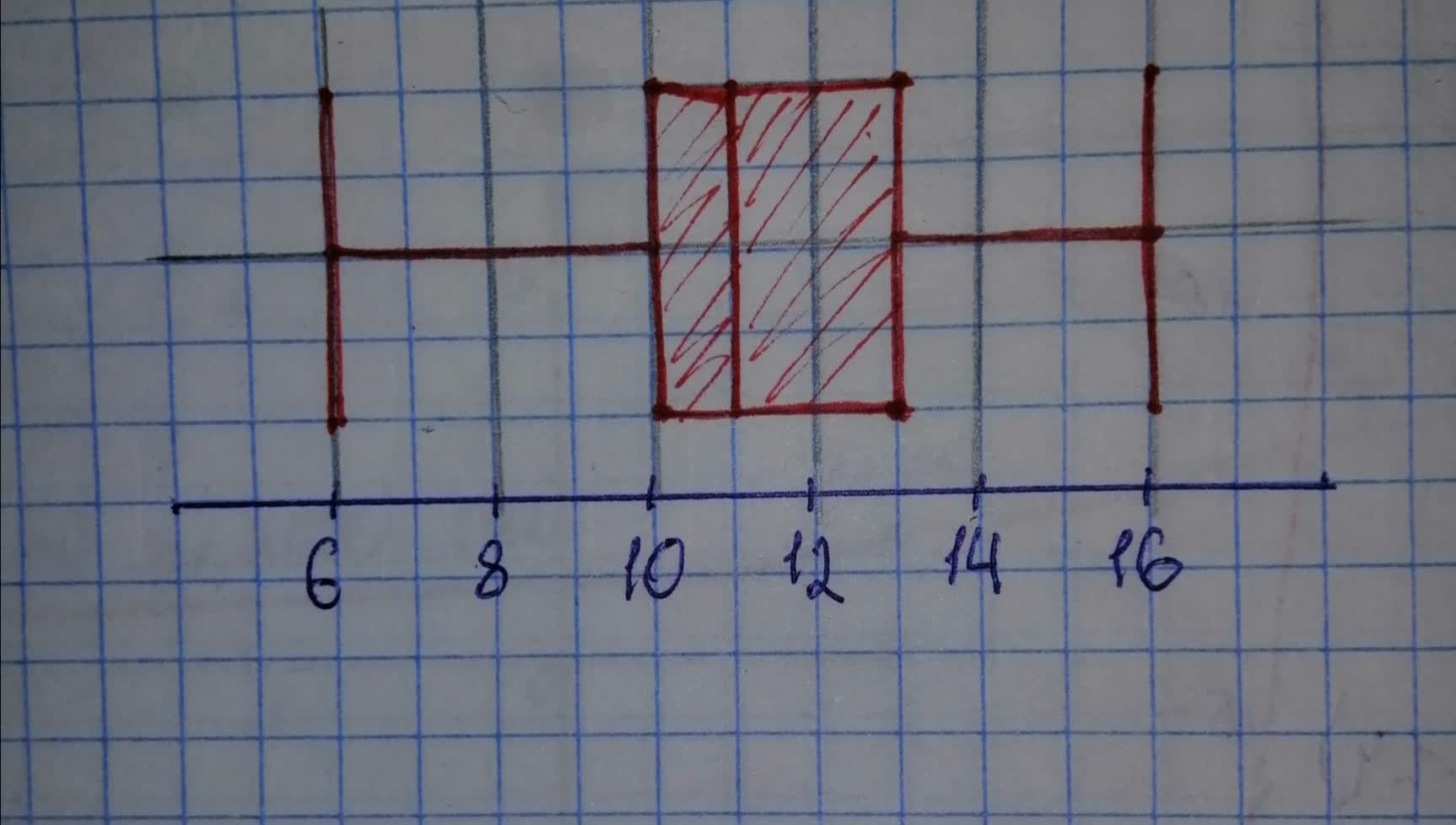# The pathogen Phytophthora capsici causes bell pepper plants to wilt and die. A research project was designated to study the effort of soil water contebanganX 2021-03-08 Answered
The pathogen Phytophthora capsici causes bell pepper plants to wilt and die. A research project was designated to study the effort of soil water content and the spread of the disease in fields of bell peppers. It is thought that too much water helps spread the disease. The fields were divided into rows and quadrants. The soil water content (percent of water by volume of soil) was determined for each plot. An important first step in such a research is to give a statistical description of the data.
You can still ask an expert for help

• Questions are typically answered in as fast as 30 minutes

Solve your problem for the price of one coffee

• Math expert for every subject
• Pay only if we can solve itgrbavit

a) Order the number from smallest to largest:

Since the number of scores is even, the median is the average of the middle scores:

The first quartile is the median of the data values below the median (or at $25\mathrm{%}$ of the data):
${Q}_{1}=10$
The third quartile is the median of the data values above the median (or at $75\mathrm{%}$ of the data):
${Q}_{3}=13$
The interquartile range IQR is the difference of the third and first quartile:

The whiskers of the boxplot are at the minimum and maximum value. The box starts at the lower quartile, end at the upper quartile and has a vertical line at the median.
The lower quartile is at $25\mathrm{%}$ of the sorted data list.
The median at $50\mathrm{%}$ and upper quartile:
at $75\mathrm{%}$b) The class width is the difference between the largest and smallest value, divided by the number of classes (round up to the nearest integer!).

Determine the midpoints, frequencies, the products of the midpoints and the frequaencies, and the products of the squared midpoints and the frequencies.
$\begin{array}{|ccccc|}\hline \text{Interval}& \text{Midpoint x}& f& xf& {x}^{2}f\\ 6-8& 7& 4& 28& 196\\ 9-11& 10& 24& 240& 2400\\ 12-14& 13& 15& 195& 2535\\ 15-17& 16& 7& 112& 1792\\ & & & & \\ & SUM& 50& 575& 6923\\ \hline\end{array}$
The sample mean is then:

The sample standard deviation is then:

Using Chebyshev's Rule with $k=2$, we know that at least

is within 2 standard deviations from the mean.